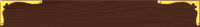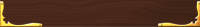## One-Dimensional Motion

Motion along a line:

• driving/walking down a street
• a ball rolling along a surface
• an object falling

Distance & Displacement
These two terms are often confused or simply interchanged.
Displacement is how far you have moved from your starting point. It doesn’t matter how many turns or reversals you have done. It is just how far you have gotten from home.

Distance is how much traveling you have done
-how far your wheels have rolled along the ground
-how long is the line tracing your path

 To keep them straight: Distance goes with Speed. These are normal everyday terms. In your car, your speedometer measures your speed and your odometer measures your distance. Displacement goes with Velocity. These are words used less commonly and are somewhat stuffy- like Science-speak. Displacement and velocity have the added factor of direction.

It is important to establish one direction as (+) and one as (-).
It doesn’t matter which way each one is as long as you stay consistent during the entire problem you are solving. Two ways to do this:

• Keep (+) to the right in the traditional way a number line is laid out
• Keep (+) as the direction of your initial movement in the problem

## Motion Graphs

Describing the motion of an object is occasionally hard to do with words. Sometimes graphs help make motion easier to picture, and therefore understand.

Remember:

• Motion is a change in position measured by distance and time.
• Speed tells us the rate at which an object moves.
• Velocity tells the speed and direction of a moving object.
• Acceleration tells us the rate speed or direction changes.
•## Worksheets

Compatability Pack for converting docx files
to doc files

Displacement vs Time 1 & 2 Worksheets

Displacement vs Time 3 Worksheet

Getting Speed vs T graphs from D vs T worksheet

Speed Time Graphs (Albert, Bob and Charlie)

Equations of Motion 1 worksheet: v = d/t

Equations of Motion 2 worksheet: a = Δv/t

Equations of Motion 3 worksheet: v = at

Equations of Motion worksheet 4: d = vit + ½at2 where vi = 0

Equations of Motion worksheet 5: d = vit + ½at2

Equations of Motion 6 worksheet: vf2 = vi2 +2ad where vi = 0

Equations of Motion 7 worksheet: vf2 = vi2 +2ad

Equations of Motion worksheet 8 : "Weapons of Math Destruction"

All Motion Equation Problems worksheet 1## Distance-Time Graphs

Plotting distance against time can tell you a lot about motion. Let's look at the axes:

Time is always plotted on the X-axis (bottom of the graph).
The further to the right on the axis, the longer the time from the start.

Distance is plotted on the Y-axis (side of the graph).
The higher up the graph, the further from the start.

##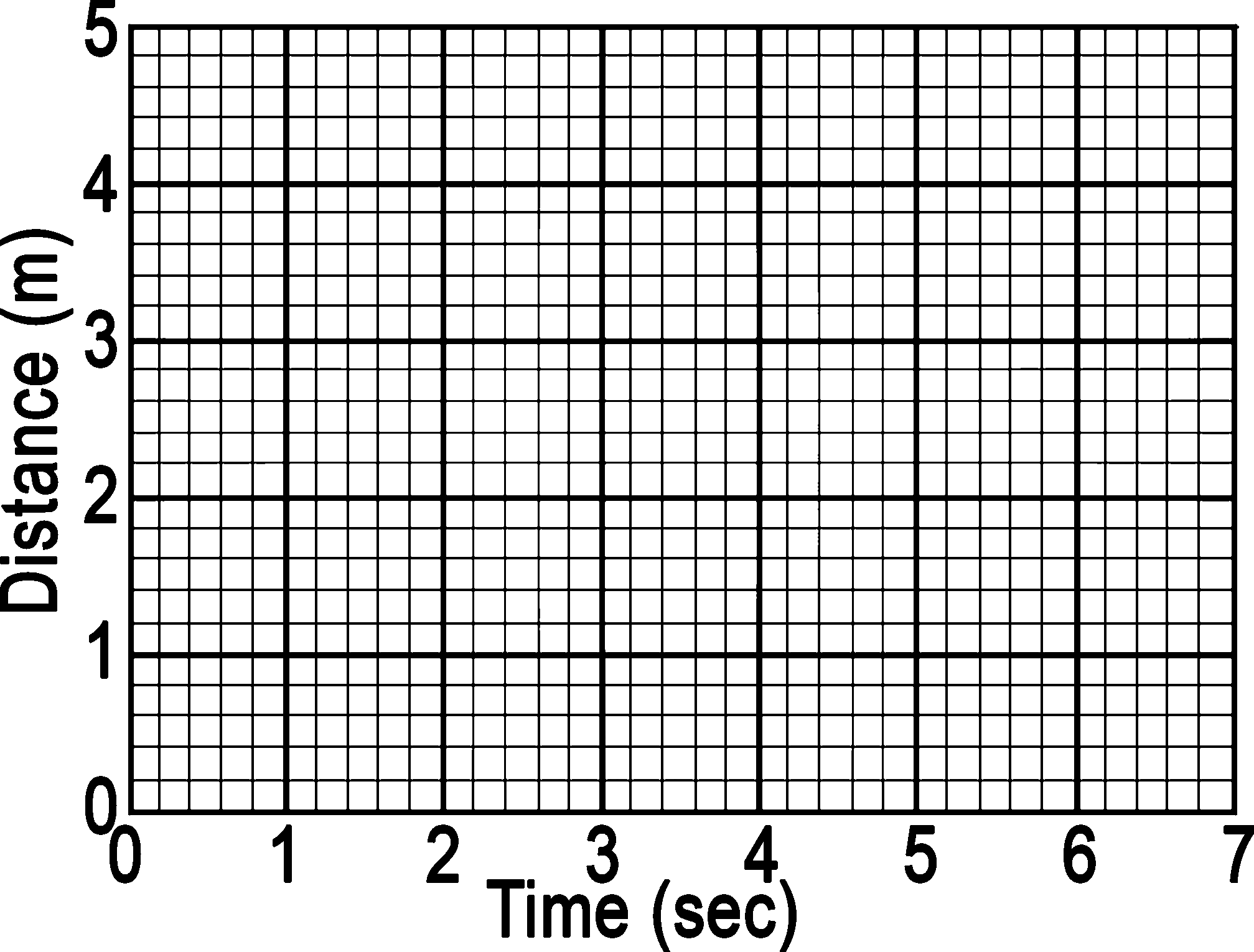If an object is NOT moving, a horizontal line is shown on a distance-time graph.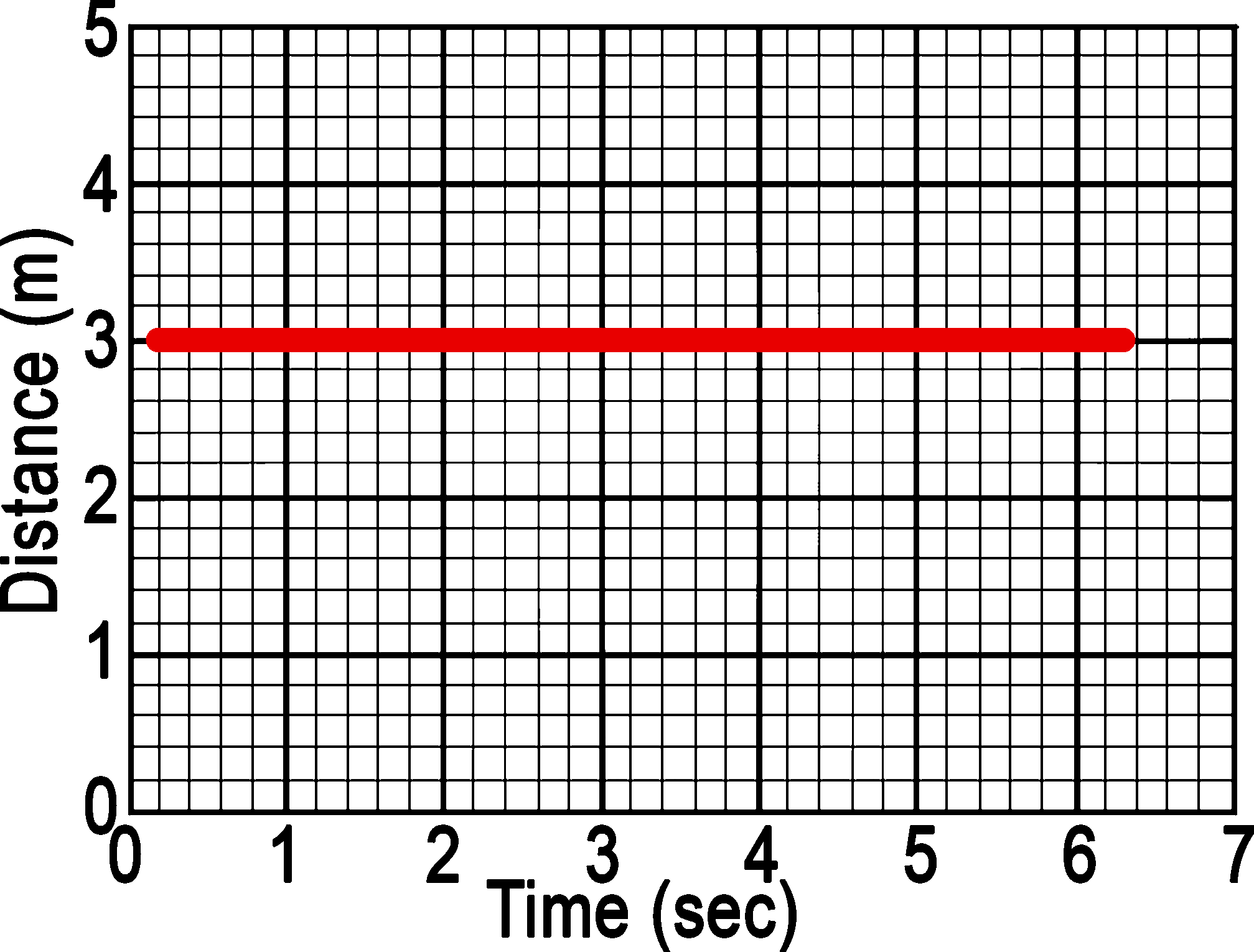Time is increasing to the right, but the distance does not change.
At one second its distance is 3 meters.
At two seconds its distance is 3 meters.
At three seconds its distance is 3 meters.
At four seconds its distance is 3 meters.
It stays at 3 meters.

It is not moving.
We say it is At Rest.

If an object is moving at a constant speed, it means it has the same increase in distance in
a given time- it will be a straight line. The slope of the line will indicate how quickly the object moved.

If an object is moving at a constant speed, it means it has the same increase in distance in a given time:
Time is increasing to the right, and distance is increasing constantly with time. The object moves at a constant speed.
Constant speed is shown by straight lines on a graph.

Let’s look at three moving objects A, B, and C: All of the lines in the graph show that each object
moved the same distance (4m), but A got there before the others: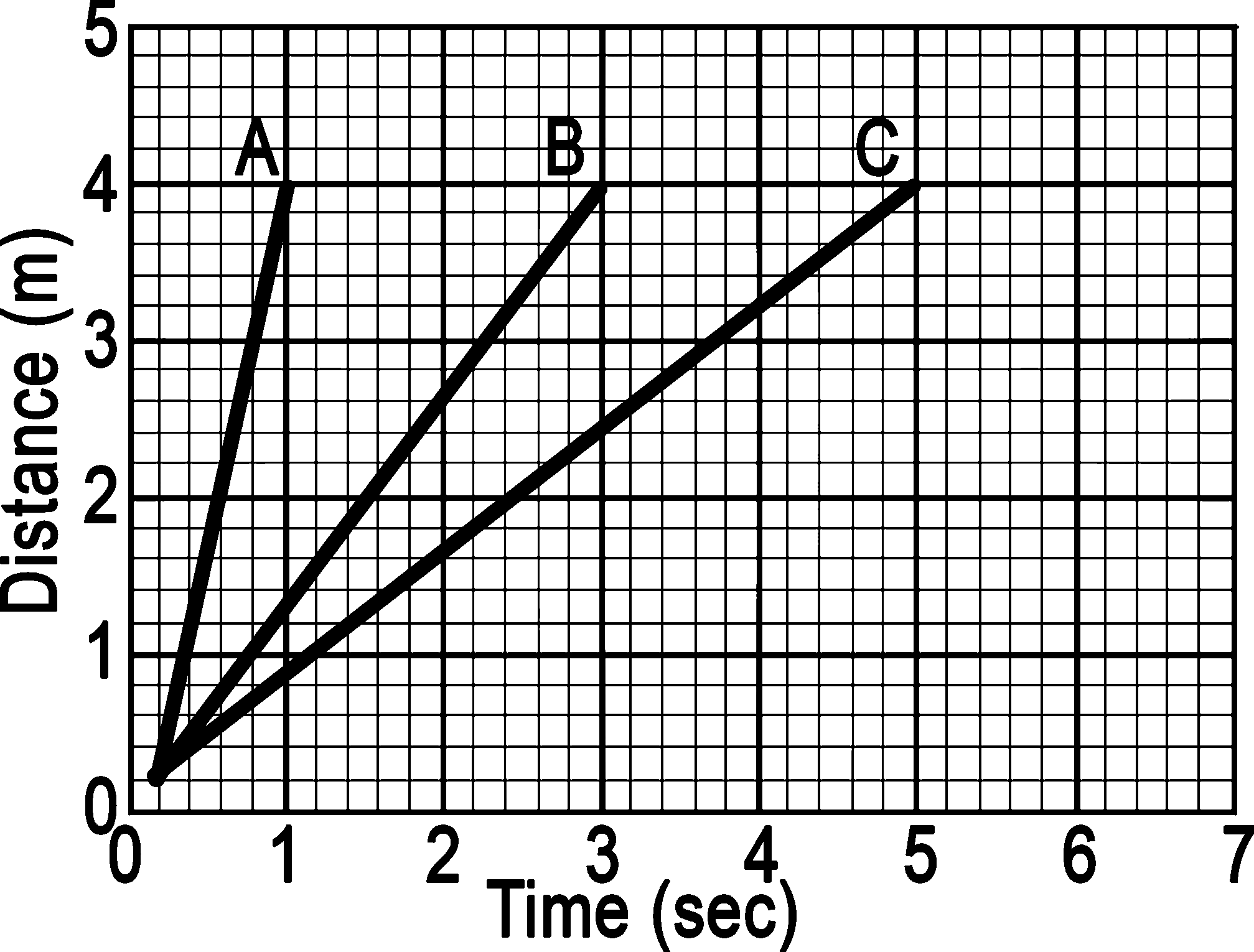A steeper line indicates a larger distance moved in a given time.
In other words, higher speed.

All lines are straight, so both speeds are constant.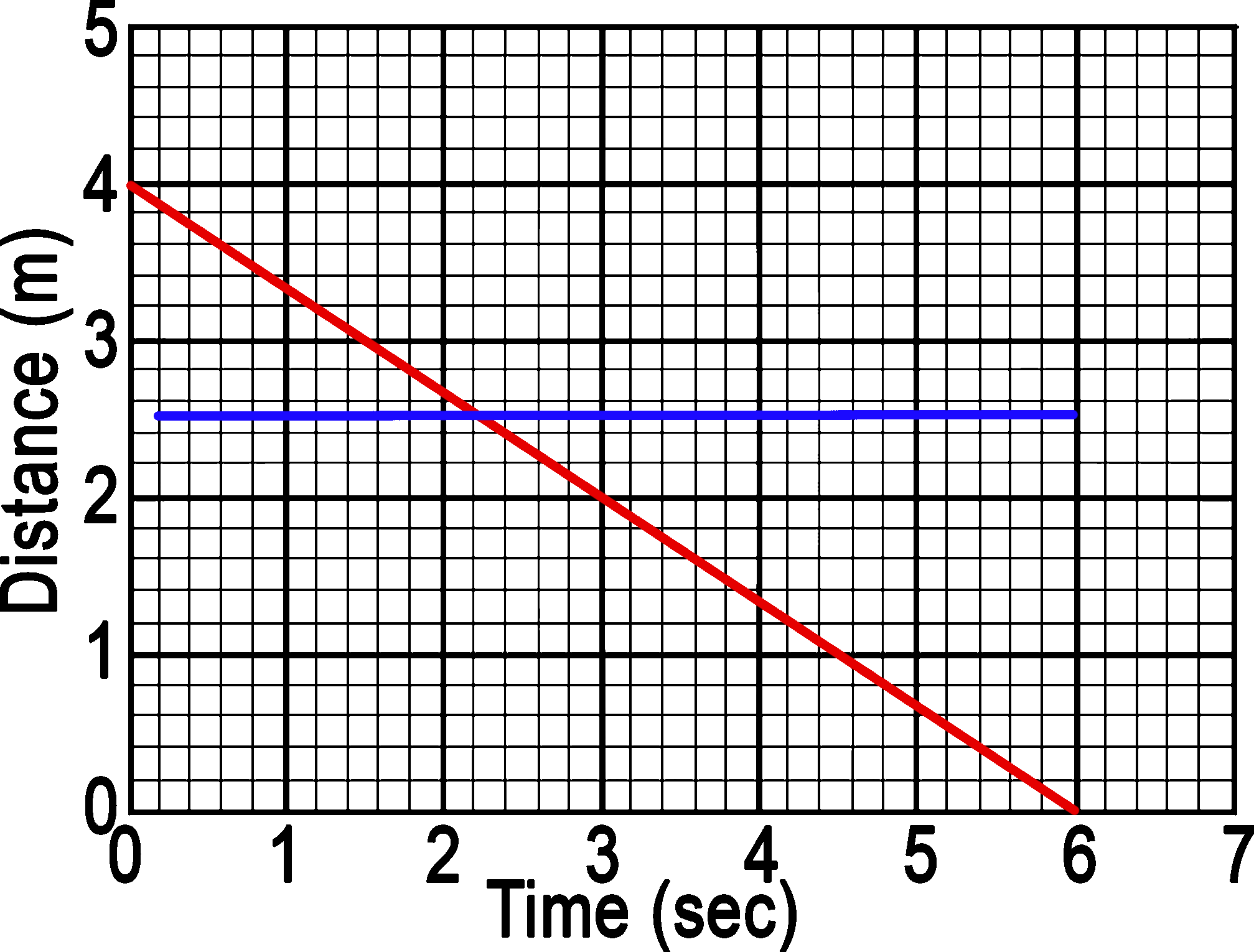Constant speed can also be “at rest” (blue line) or a “negative velocity” (red).

Graphs that show acceleration look different from those that show constant speed.

The line on the graph below is curving upwards. This shows an increase in speed, since the line is getting steeper:

In other words, in a given time, the distance the object moves is changing (getting larger). It is accelerating.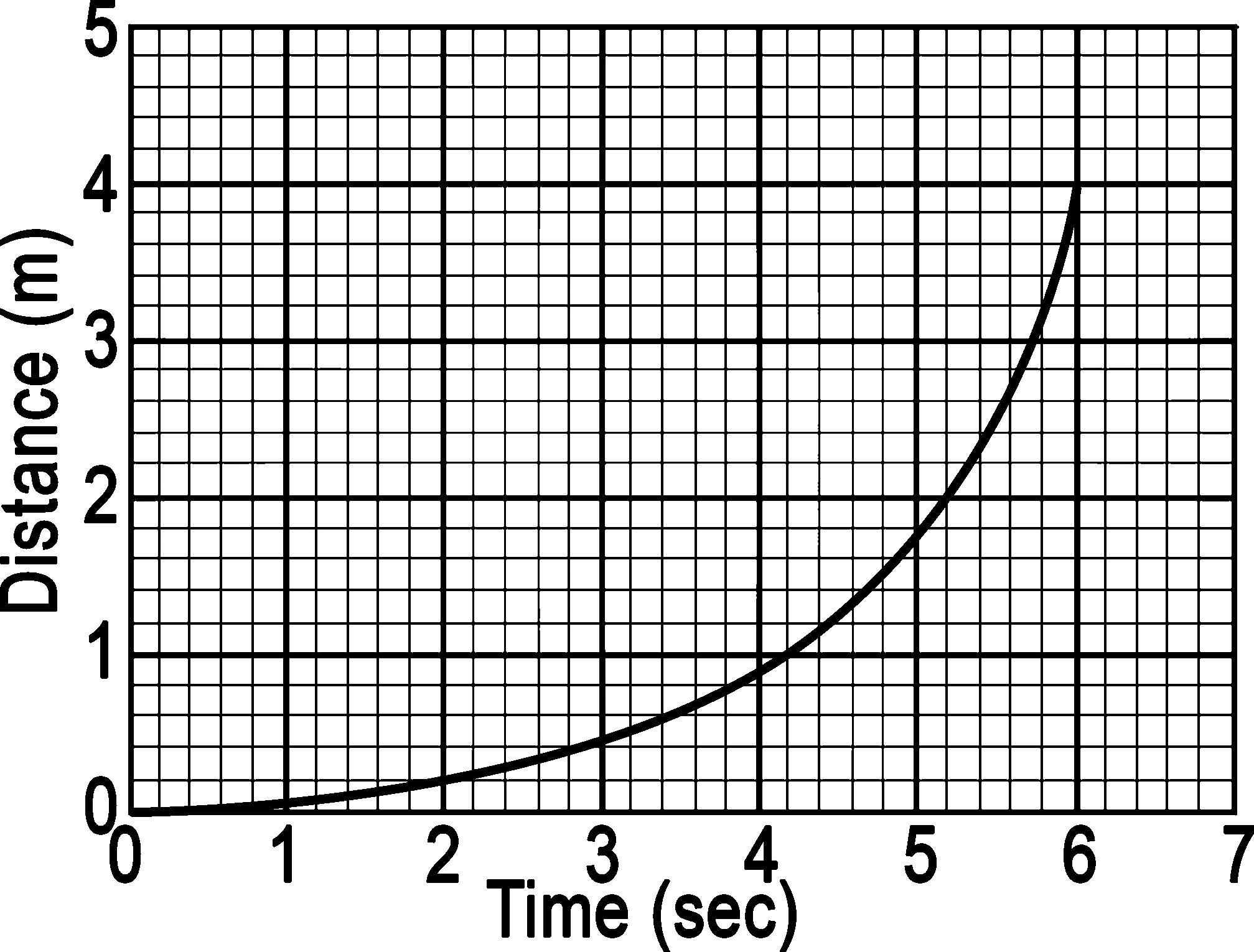Graphs Summary:

A distance-time graph tells us how far an object has moved with time.

• The steeper the graph, the faster the motion.
• A horizontal line means the object is not changing its position - it is not moving,
it is at rest.
• A downward sloping line means the object is returning to the start.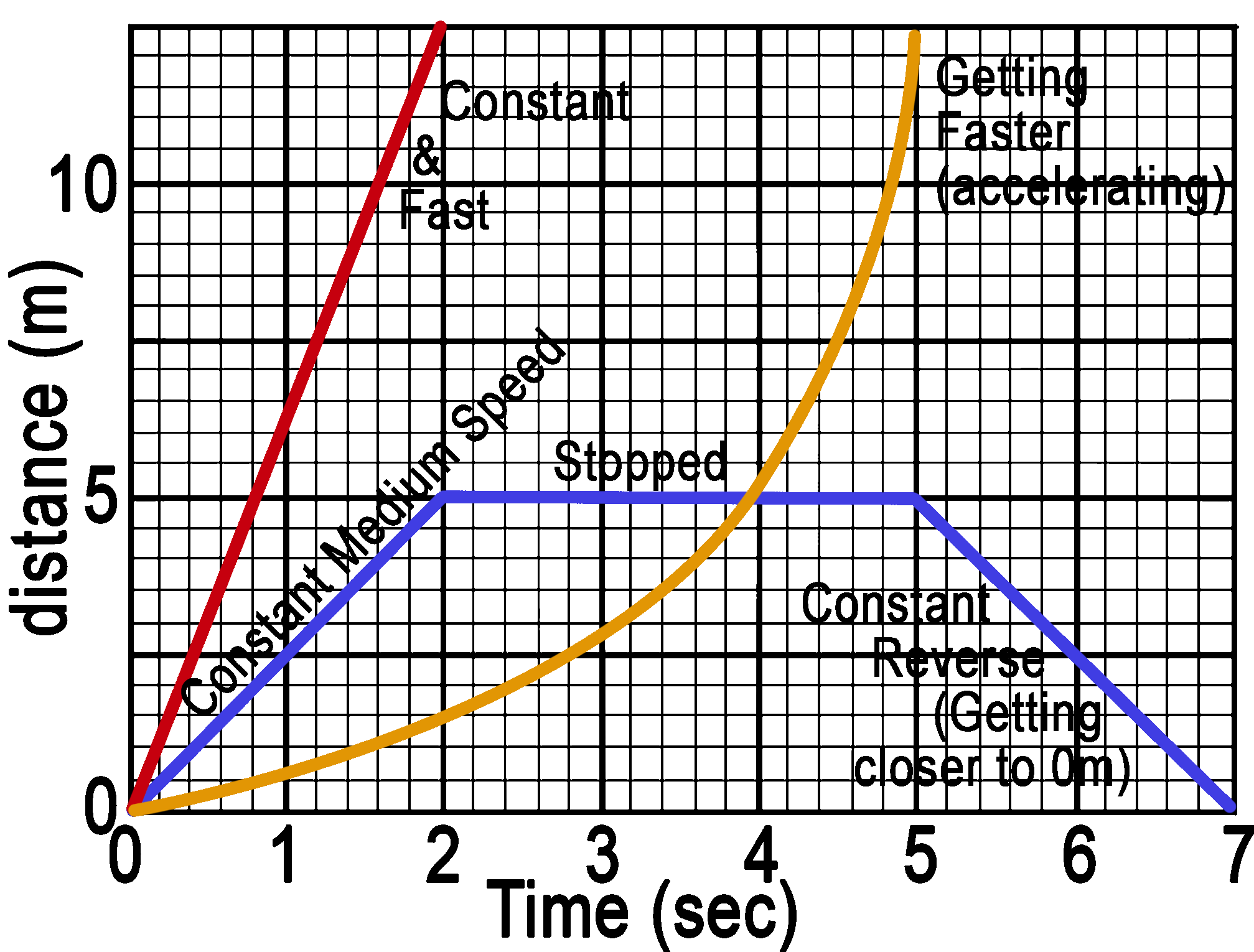A great online review game of motion graphing.
Motion graphing review:
http://www.theuniverseandmore.com/

## Average Speed vs Instantaneous Speed

Average speed is the averaged speed of an object between point A and B based strictly on the distance traveled and the time it took. It doesn’t matter mathematically if the object was traveling at different speeds during that duration.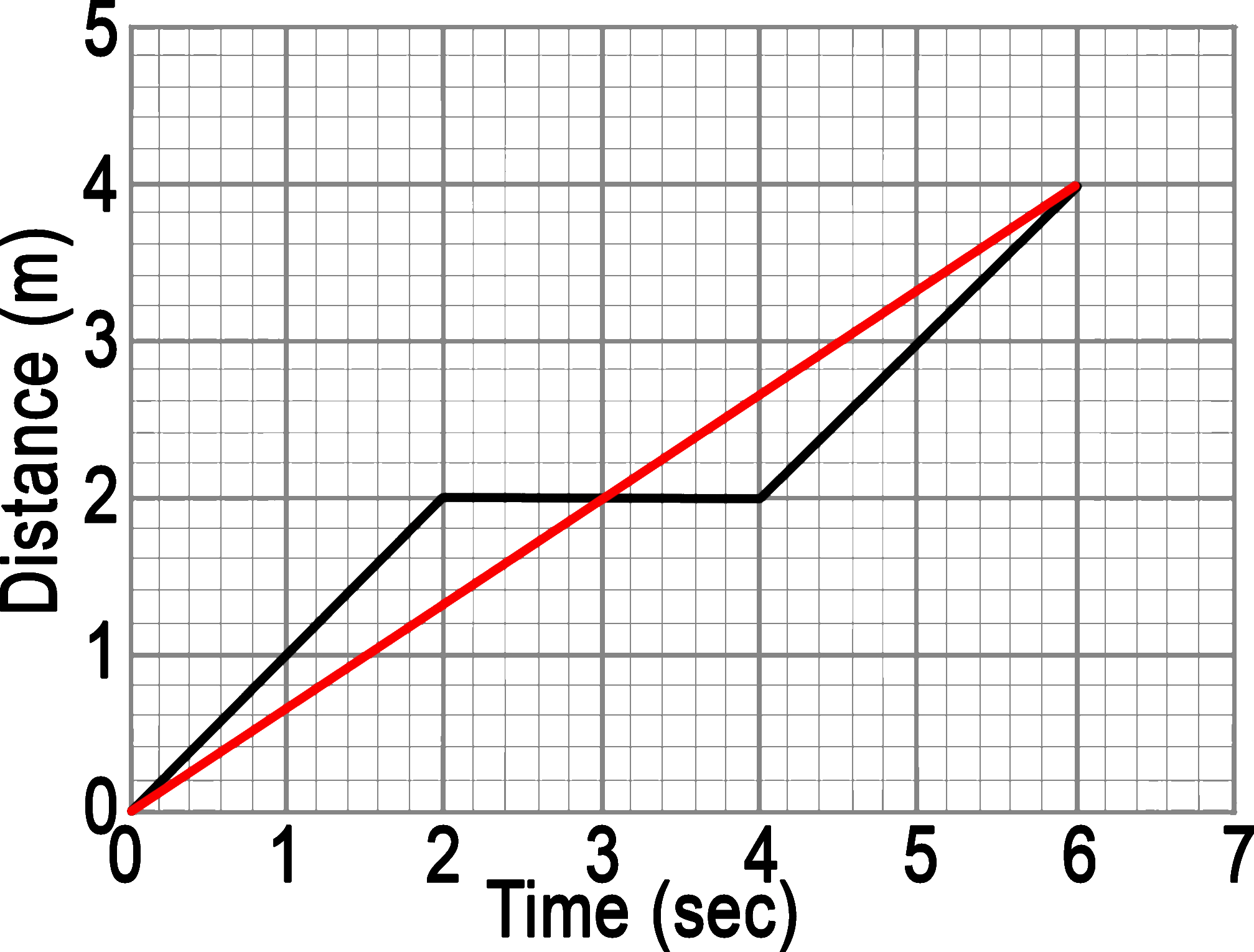Consider this: in the graph above, Blackie the cat pounces, stops and pounces again as shown by the black line above. Even though Blackie pounces quickly at 1 meter for every second, if you throw in the time she was stopped it averages out to .67m/sec (4m/6sec).

Ole Red the bloodhound, sniffs along at a steady .67 meters every second.

Averaged over the entire 6 seconds, both animals have an average speed of .67m/sec.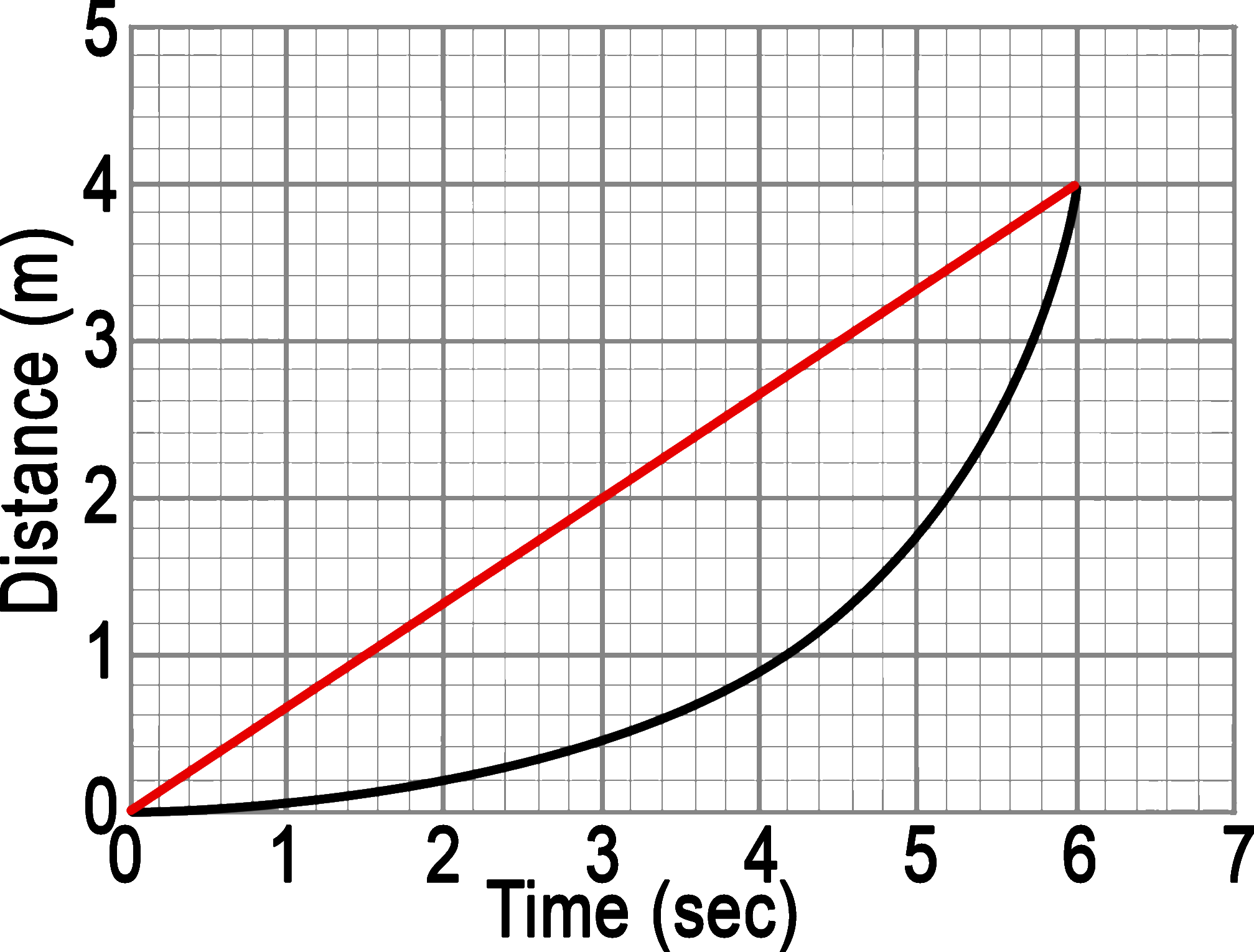But! If the object is changing its speed, for example constant acceleration as shown above, we run into a problem.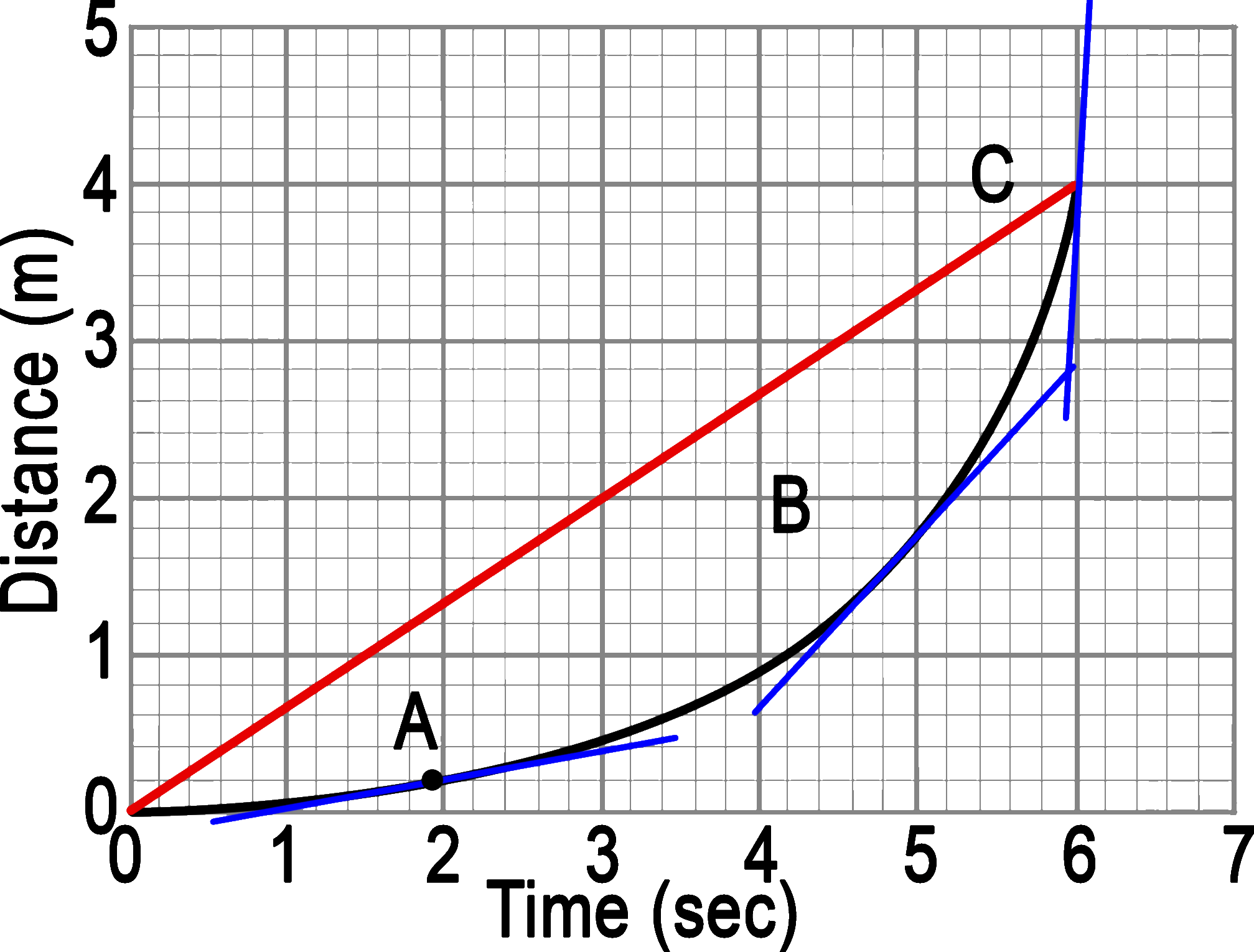In the graph above (speed 4), the average speed is .67m/sec (the red line), but you can see that the actual speed is constantly changing. At the beginning: A, the speed is very slow (gentle gradient). Then the speed increases until, at the end: C, it is moving very fast (steep gradient). If we wanted to get the instantaneous speed at A all we would need to do is to get Δd/Δt. But at the exact point A there is no change in distance or speed (it is a point). The only way to get a speed is to measure a CHANGE in position over a CHANGE of time. The trade-off is that the bigger the time sampled, the greater the difference between the instant speed and the average speed can become. See the difference between the average slope (.67m/sec) and that of A (.2m/sec)? The smaller the time sampled the closer the two values get to zero and any inaccuracies due to measurement become more significant.

There is a rule in Science called the Heisenberg Uncertainty Principle that says that you cannot know the EXACT speed AND position of an object. You can exactly know only one of them. For example, if you want to know the exact speed of a moving particle, you must let it travel some distance in order to measure its change in position. The best way to work with this principle would be to get both quantities with a good enough precision to do what you need. Allow the object to move just enough of a distance so that you can measure how much time it takes to move.

## Interpreting Graphs

The two charts below are based on this worksheet:
Graphing Motion 1 & 2 Worksheets

 Each point on the graph shows the object’s location. Since v= d/t, slope of this graph is the velocity Average velocity is the slope between the two points being averaged Instantaneous speed of a point is the slope of that section on line A flat line is at rest Sloping upwards is a positive velocity (going forward) Sloping down is a negative velocity (going in reverse) An up-curving line is increasing its velocity (accelerating) A down-curving line is decreasing its velocity (accelerating) Total distance traveled is the sum of all the ups and downs Total displacement is adding all the (+) and (-) ups and downs.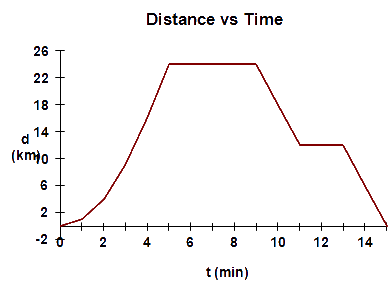Flat lines represent a constant, steady speed Lines sloping up are positive acceleration- going faster Lines sloping down are decelerating (slowing down) The slope of these lines are the acceleration. Distance traveled is the area under the graph or section of the curve.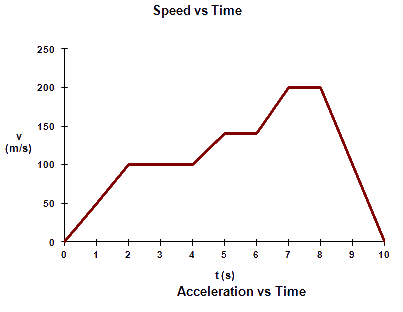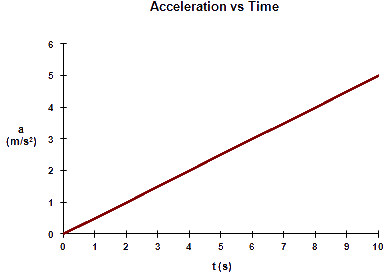• Acceleration is a measure of how hard you press on the gas pedal
• Velocity is the area under the graph
• This graph shows that you are pressing the pedal harder and harder.
 Note: assume all examples, discussions and problems are without friction and air resistance. There are equations that take these forms of friction into account, but in order to learn the basics, we must start simple and under ideal circumstances.

Uniform Motion Lab (motion with one speed)- A "bully cart" is used to drag spark tape through a spark timer to collect distance and time data for a constant velocity cart.

## Ways to keep points on Labs:

• Reduce fractions and radicals to a decimal
• Use correct or at least approximate sig. figs.
• Keep Math organized
• Use full sentences
• Avoid starting answers with “because”
• No short cuts:
• b/c
• 'cause
• lol
• Using “it” without defining what “it” is
• “it will increase”
• Acceleration will increase.
• Write the equation you are using
• Substitute with values
• Show all Mathwork
• Graph in pencil
• Units for all problems
• Throughout the problem
• Have a meaningful conclusion including
• what the lab was used to demonstrate
• what did the lab show
• explanation of any relationships or graphs

## Getting Distance from a velocity vs time graph (Area under the line)

Using the graph below, find the distance that the ball has rolled in 5 seconds while moving at 10m/sec.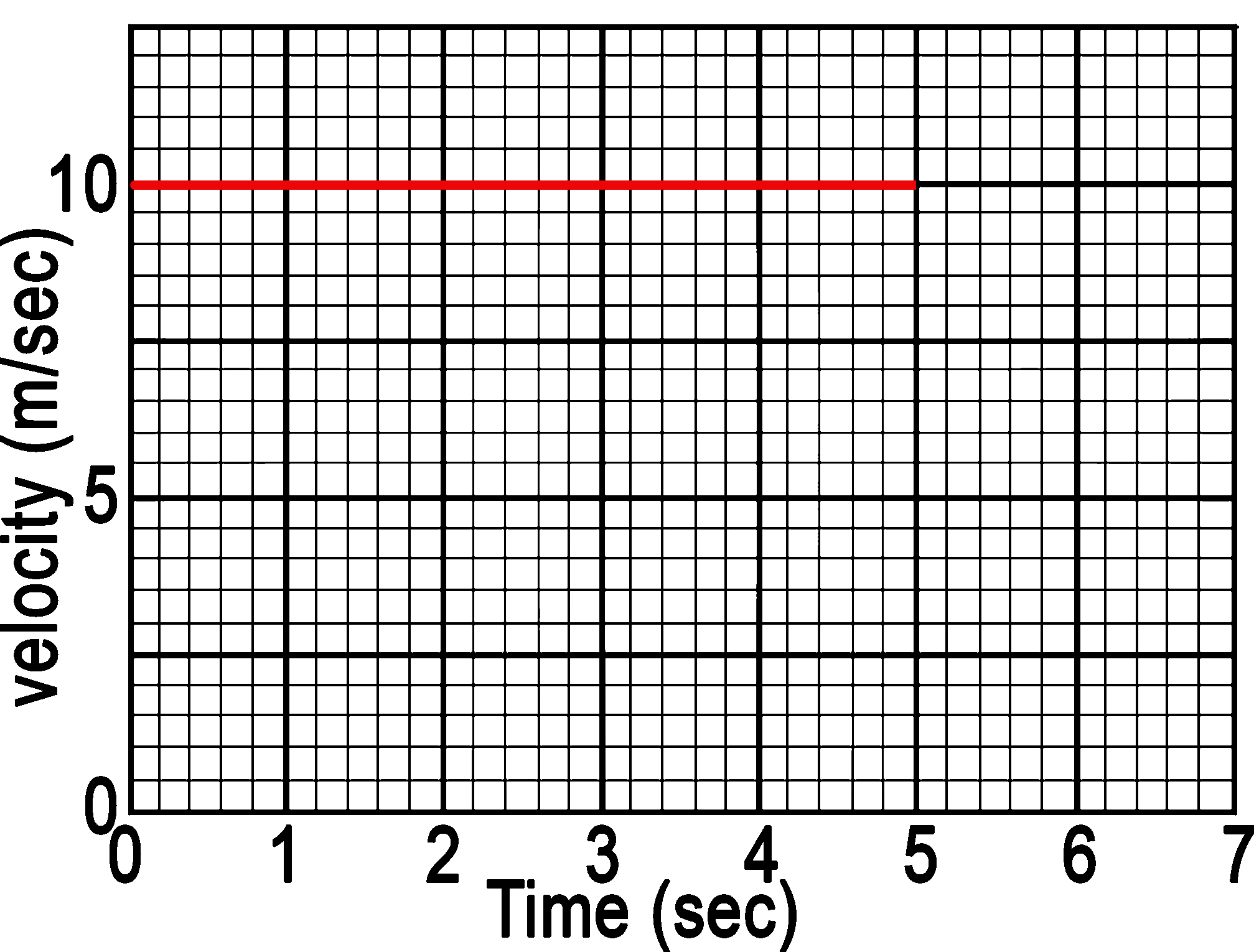Every second that the ball rolls, it travels 10m (hence 10m for every second). After one second it has traveled 10m which is the area under the 1st second of the graph.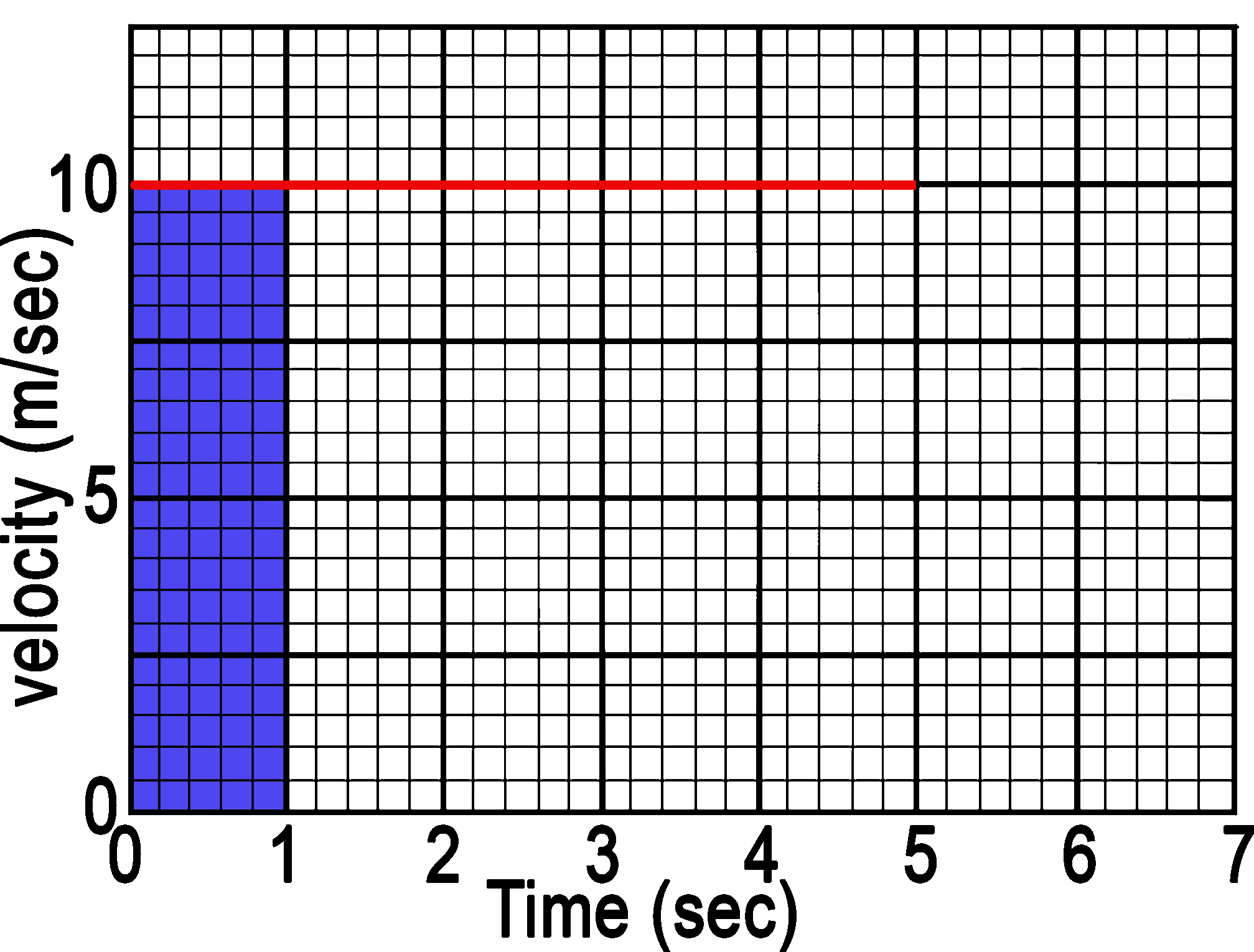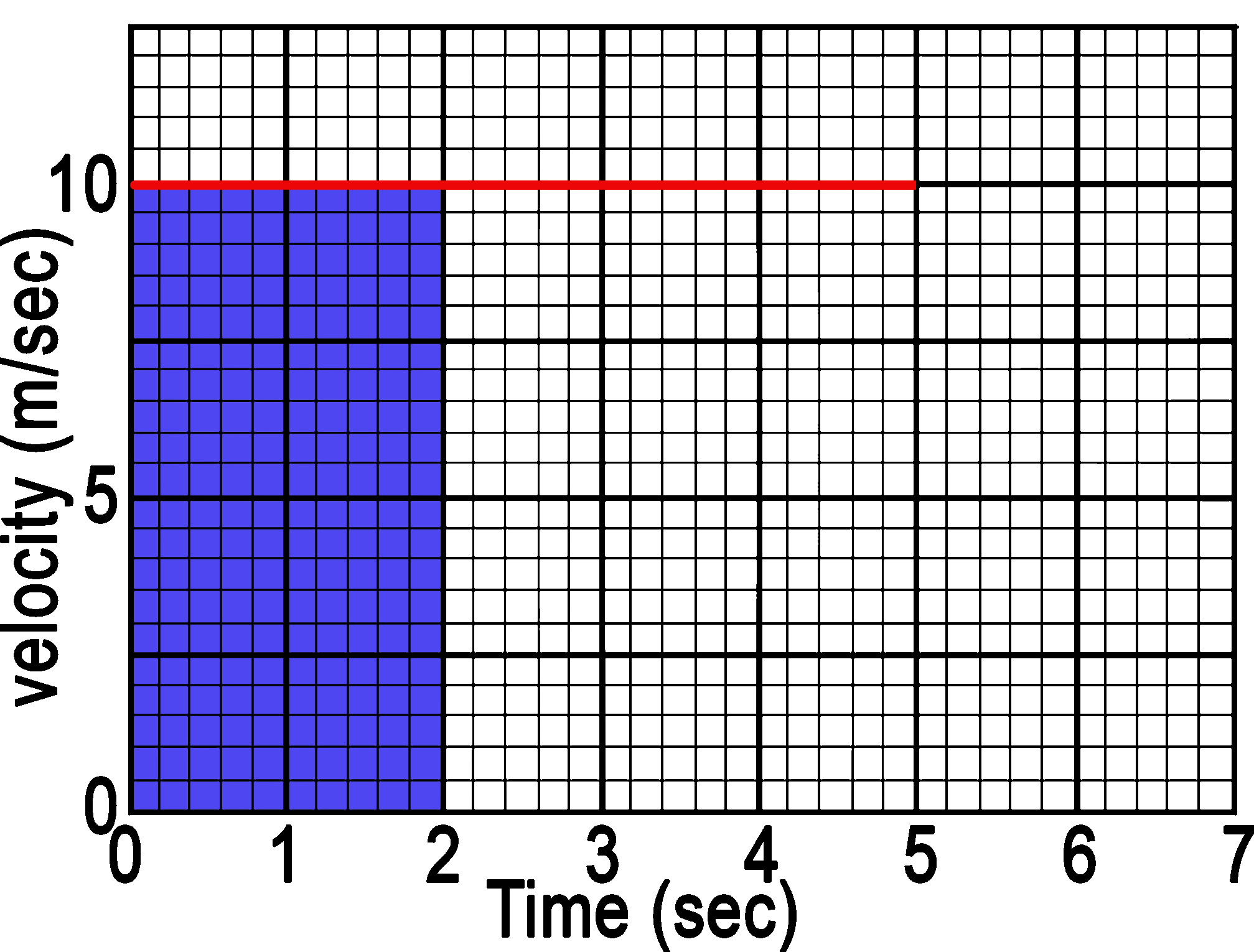After 2 seconds the ball has traveled 20m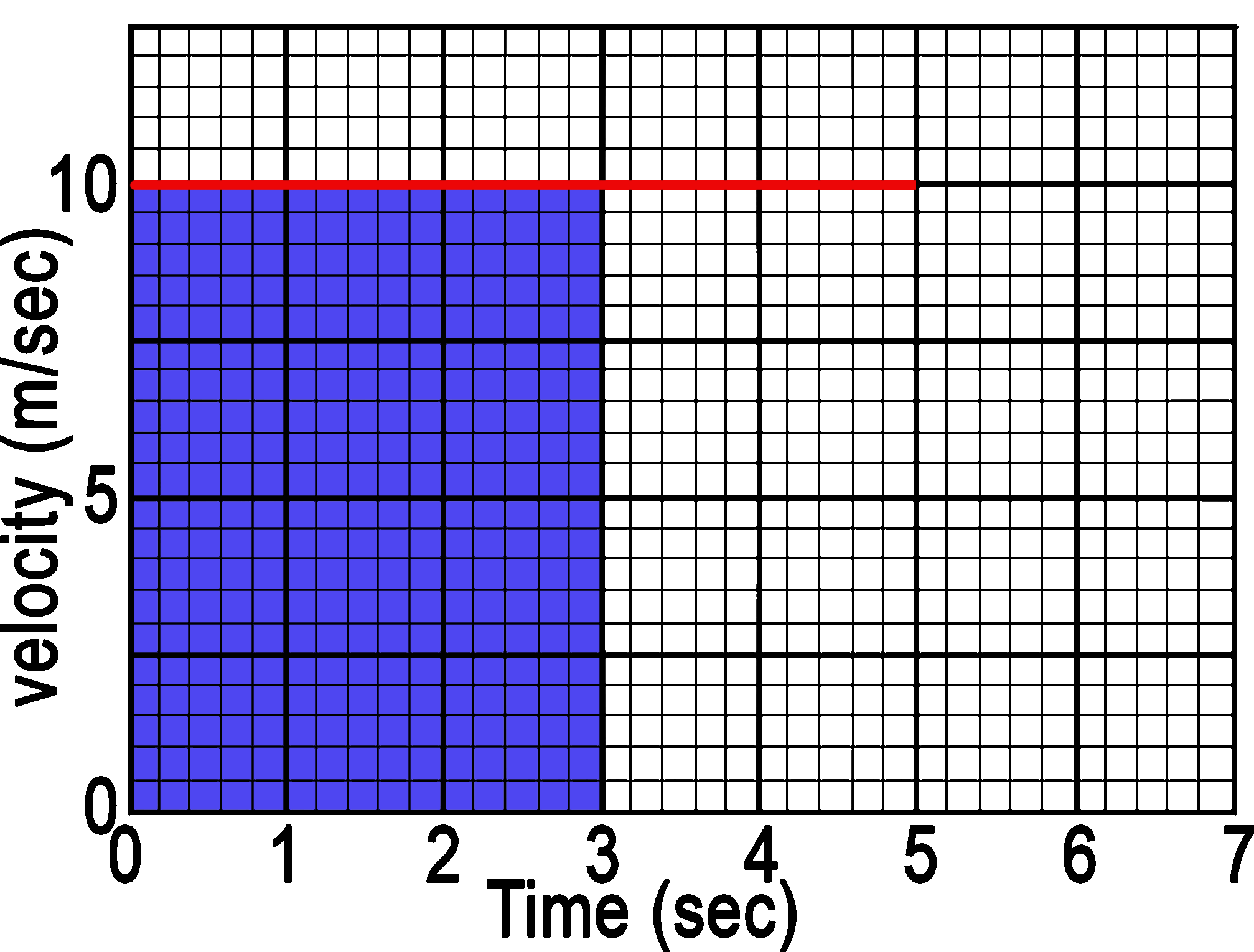After 3, 30m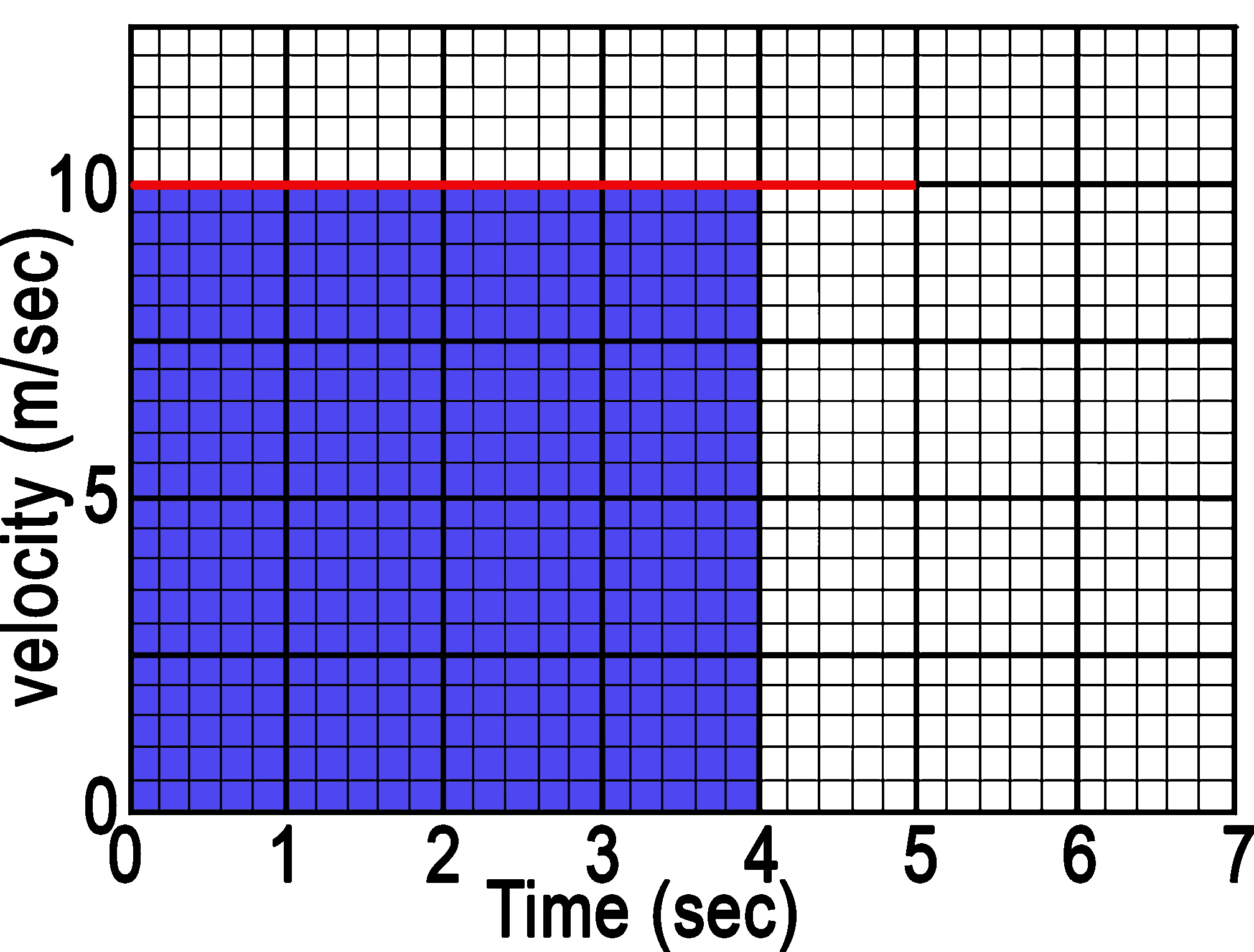After 4, 40m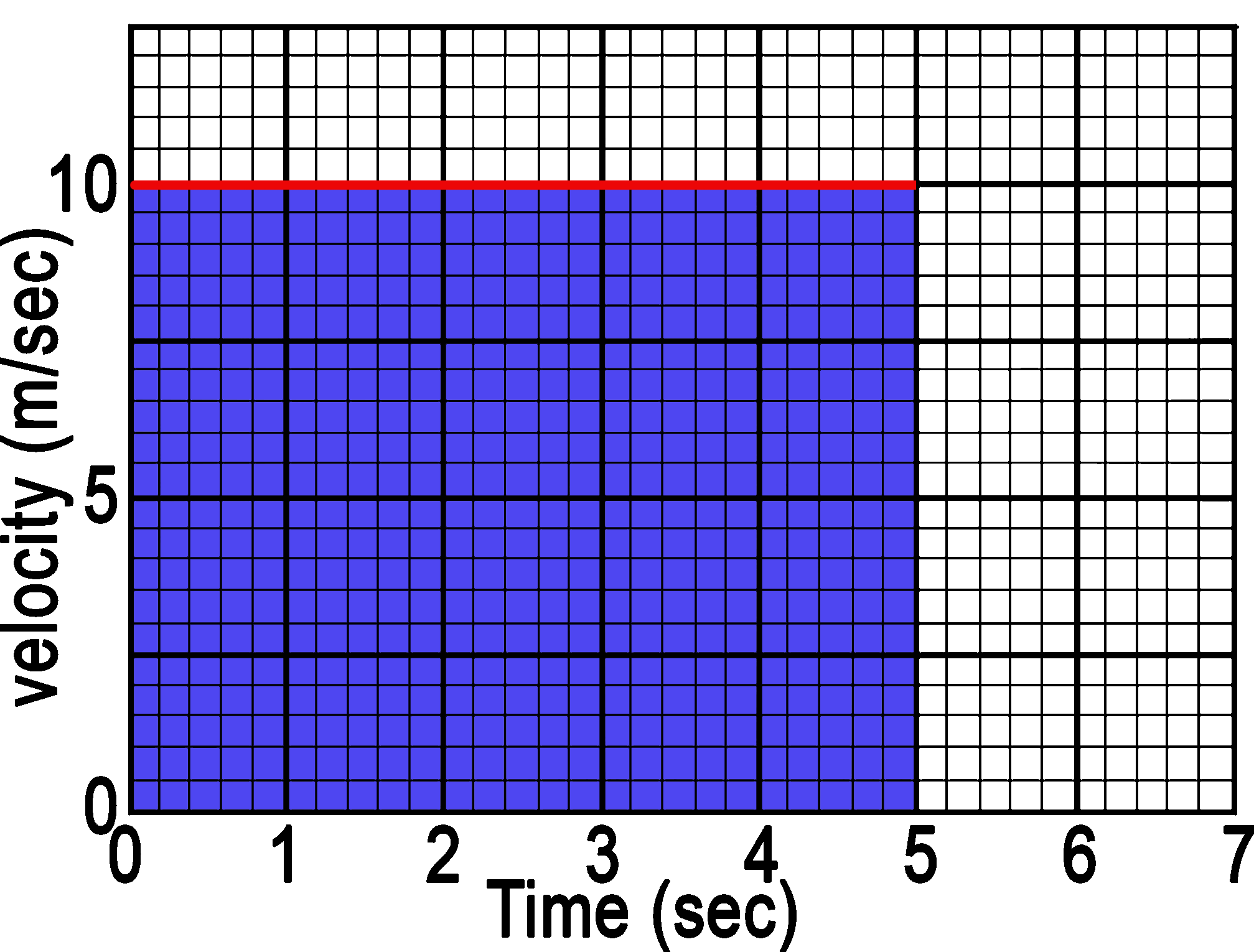And after 5 seconds it has traveled 50m

If the line is not a horizontal line (representing a constant velocity) then break it up into shapes with areas that you can calculate. More complicated shapes such as curves require calculus.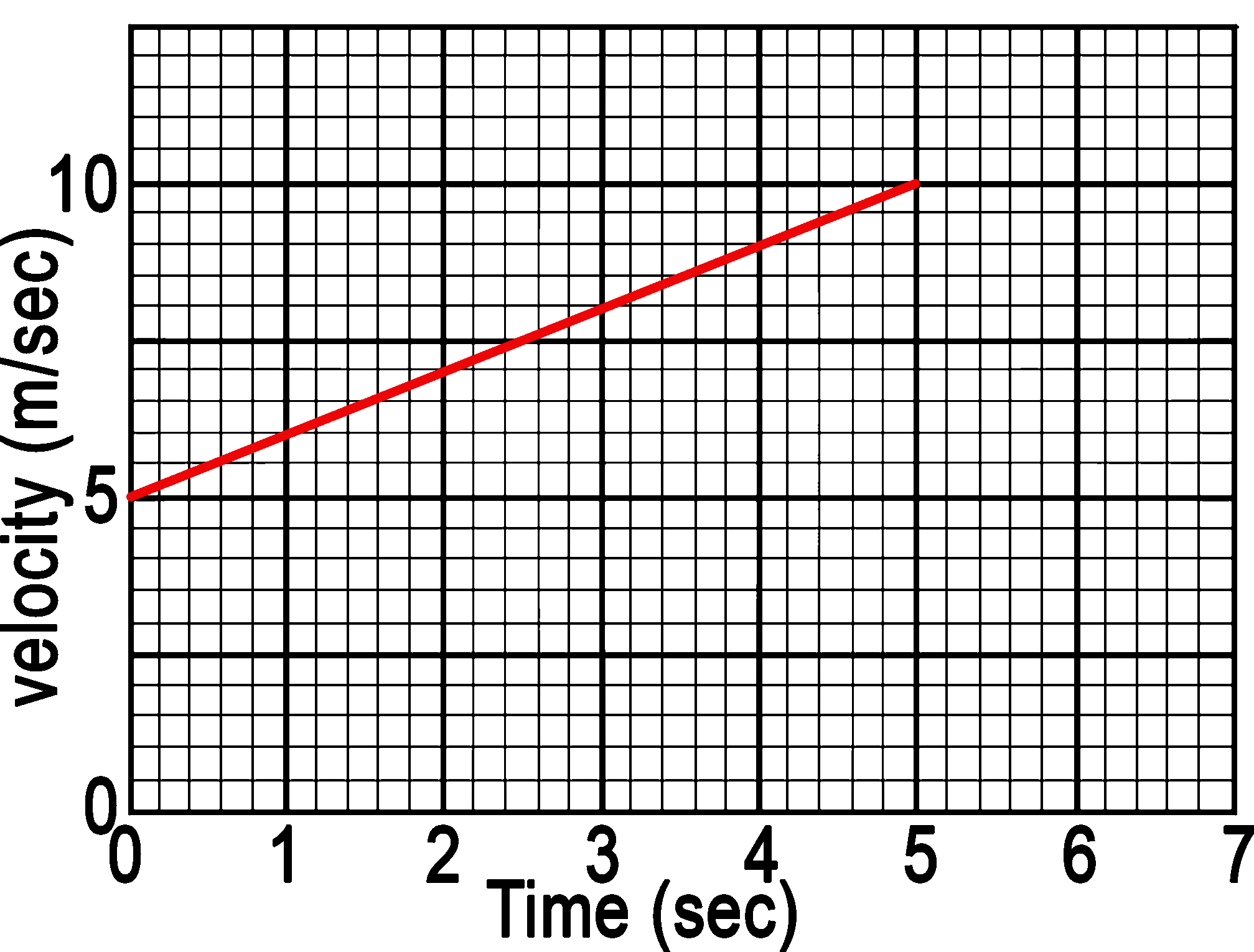In this case the area under a diagonal line can be broken into a rectangle and a right triangle.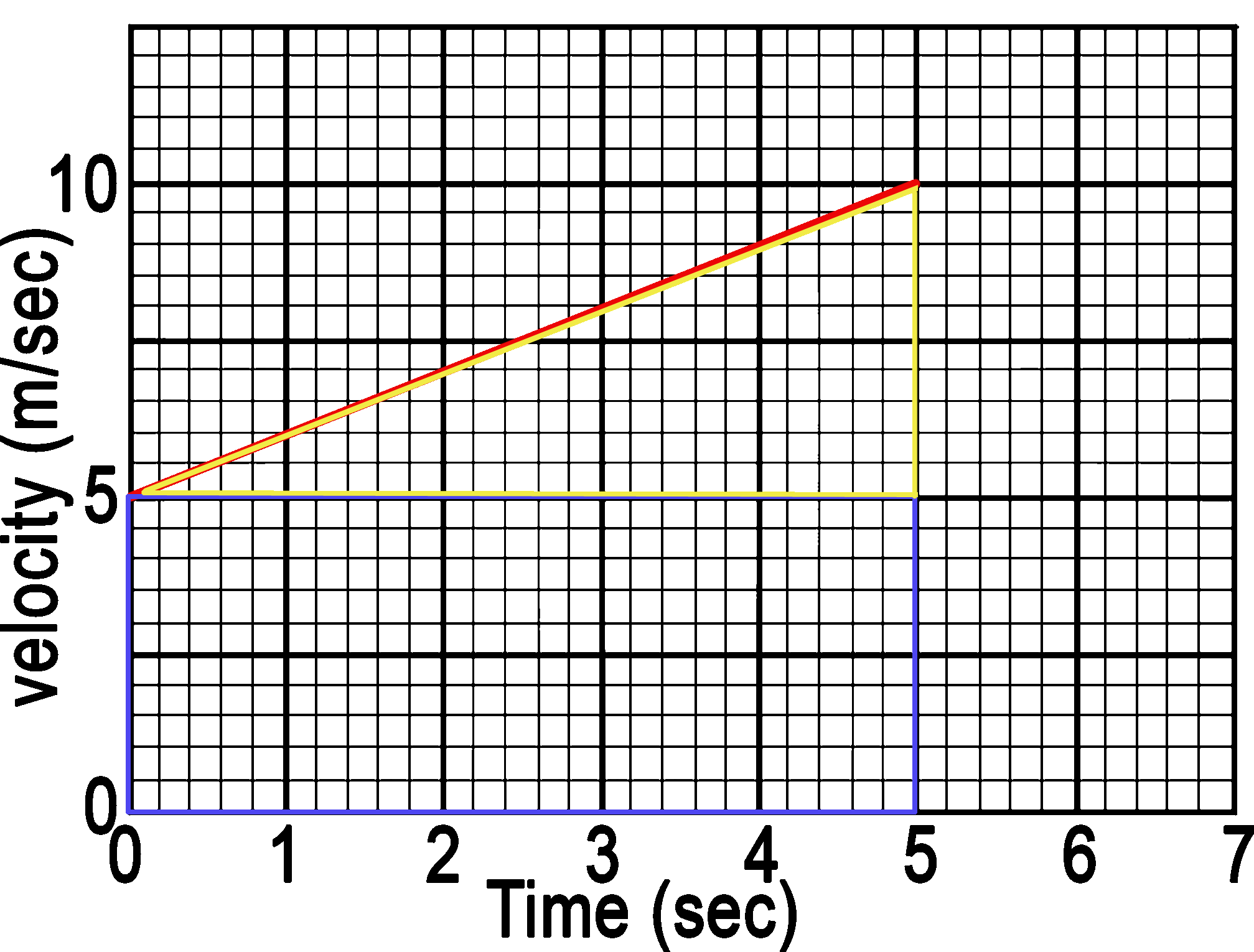The area of the rectangle is base x height or, in this case velocity x time. The area of the triangle is ½ base x height, or, ½ velocity x time.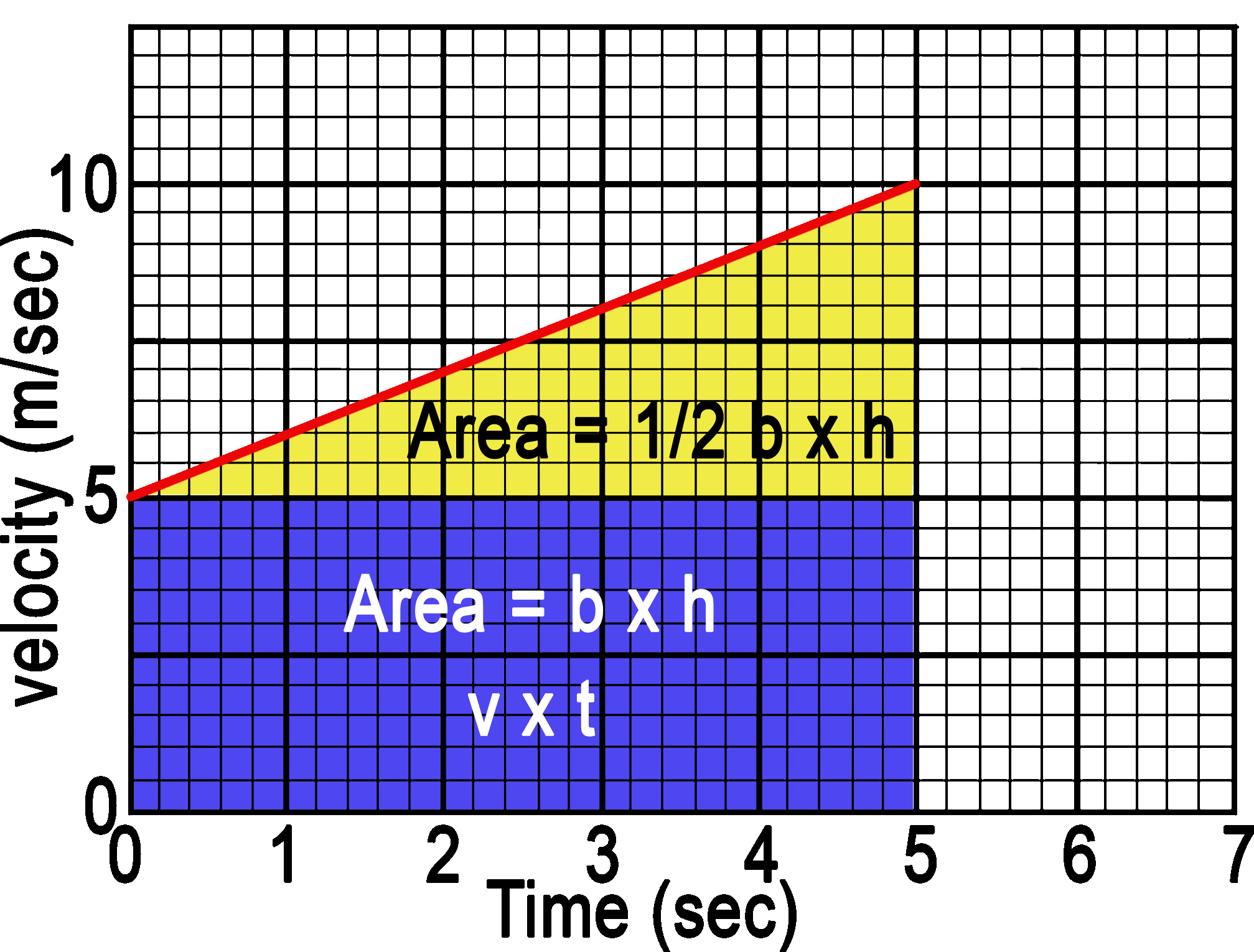5m/sec x 5sec = 25m +½ x 5m/s x 5sec = 12.5m Total area is 37.5m

Average Velocity Problem: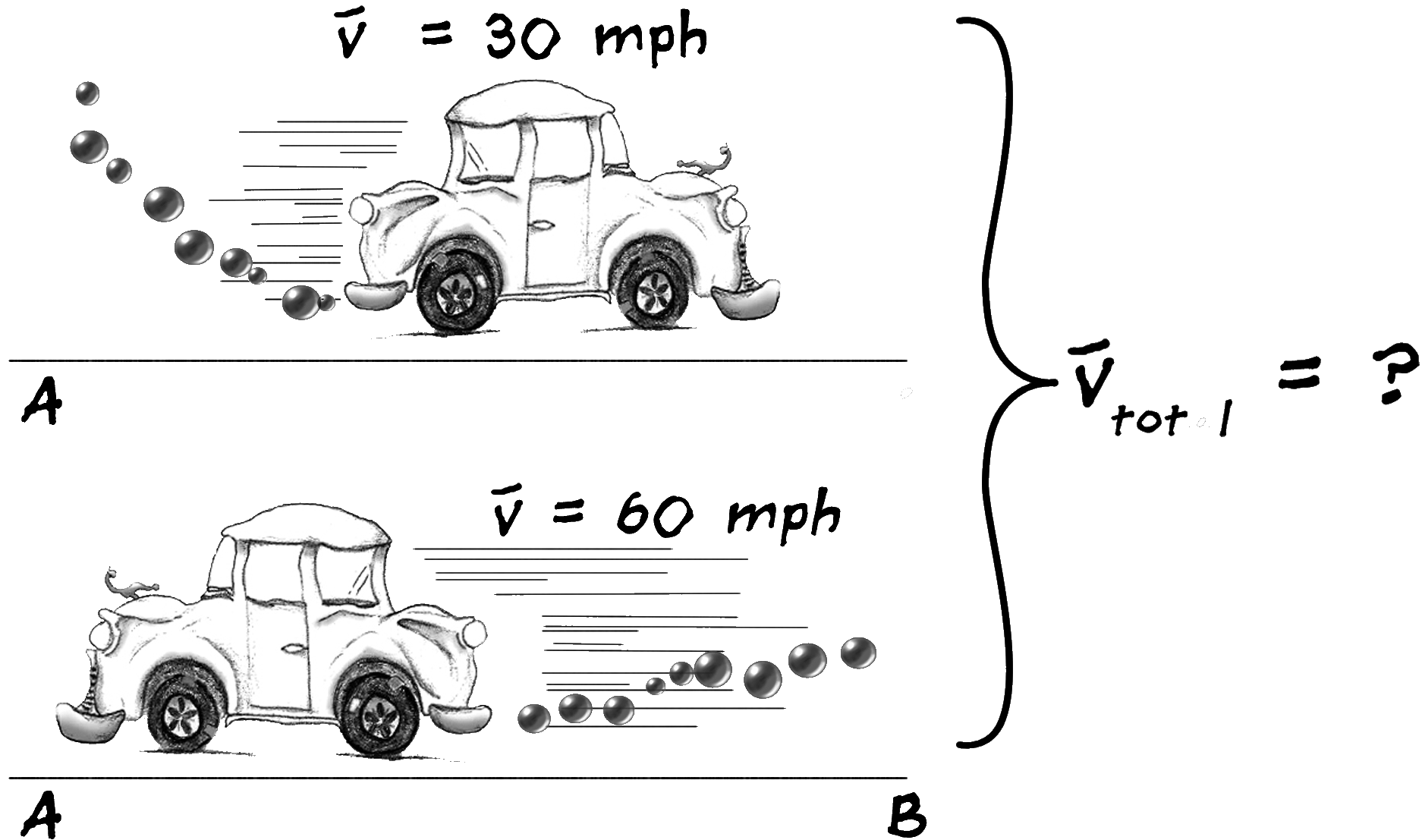Suppose in making a round trip you travel at uniform speed of 30 mph from A to B
which are separated by 100 miles, and return from B to A at a uniform rate of 60 mph.
What would be your average speed for the round trip? (Careful!)

## Equations of Motion

• v = d/t: you already know
• a = Δv/t
Acceleration is a change in velocity
Speeding up
Slowing down: AKA decelerating or a “negative acceleration”
Changing direction (more on that MUCH later)
Units are m/sec2
a = Δv/t problem:

A car goes from 0 m/s to 160 m/s in 5 seconds
What is the acceleration?

a = (160m/s - 0m/s)/5sec
a = 32m/s2

### How can you square a second?

It seems strange to have seconds "squared" since it is an intangible quantity. If it helps, 32m/s2 can also be read as 32 meters per second per second, which means

“for every second the velocity changes by 32m/s”.

Equations of Motion worksheet 1: v = d/t

Equations of Motion worksheet 2: a = Δv/t

## Acceleration due to Gravity Lab

• The Spark Timer uses a small spark to burn a mark onto paper tape 60 times a second.
• MAKE SURE TIMERS ARE SET TO 60Hz
• Thread a length of spark tape through the timer and then tape it onto the mass.
• When the weight falls, it will pull the tape through the timer leaving a trail
of dots at measured intervals.
• Write down the object that you drop.
• Circle the first dot that is clearly separated- this will be our zero starting point.
• Circle every 3rd dot
• At 1/60th second, every 3rd = .05 sec
• Set up the data table
 t d 0.00 0.05 0.10 0.15 0.20 ...
• Measure the distance from the “zero” dot and enter it into a data table.
• Make a d vs t graph
• Use the entire graph paper
• Work in pencil
• Connect dots with a smooth curve
• Every person makes their own
• To calculate speed:
• We want the instantaneous speed at the moment that the dot was made
rather than the average speed since the beginning.
• Measure the distance between the dots before and after your circled dot.
• This value will be called Δd
• This will give you the distance the mass dropped during the 2/60ths of
a second surrounding the dot.
• v = Δd ÷ 2/60sec. v is also more easily calculated as Δd x 30/sec
• Fill in two more columns and then graph d vs t and v vs t.
 t (sec) d(m) Δd(m) v(m/sec) 0.00 0.05 0.10 0.15 0.20 ...
• Questions to consider in your conclusion:
• How did distance traveled, Δd and velocity change through the experiment?
• How did your measured acceleration compare with those of your classmates.
• What was the correlation between mass and acceleration?
• Predict how the results would change if you doubled the weight.
• Be sure all measurements and calculations have units
• Don't forget the reason why we did the lab! What is the acceleration due to gravity? (The slope of v vs t.)

Astronaut dropping a feather and a hammer on the moon:

### vf = vi + at

Final velocity (vf) is your original velocity (vi) plus any velocity you gained from acceleration.”
It is usually v = at but the vi is added in incase you are not starting from a rest.
“v is where it’s at”

vf = vi + at problem: (actually starting at rest so it is vf = at)
What is the exit velocity of a bullet after it travels down a rifle barrel with
an acceleration of 2.0 x 105 m/s2 in a time of .003 seconds?

vf = vi + at problem (where vi is not zero):
A rocket car is cruising down a road at 40 m/s using only the conventional
engine in the car. The driver then engages the rockets and the car accelerates
at a rate of 10 m/s2 until the engines cut off after 10 seconds of thrust.
What is the final velocity?
(Professional driver on a closed course. Do not attempt)

Equations of Motion worksheet 3: v = at

### d = vit + ½at2

It is usually d = ½ at2 but you add in the vit in case you are already moving.

d = vit + ½at2 problem (starting at rest so it is d = ½at2):
A model rocket provides an acceleration of 35 m/s2 for 6 seconds.
How high does the rocket reach when the engine cuts off?

d = vit + ½at2 problem (where vi is not zero):
A Klingon Bird of Prey is hurtling through space at 250 km/sec when it is
caught in the gravitational pull of the sun. At its current position, the
Bird of Prey’s acceleration due to the Sun’s gravity is 200 m/s2.
The ship is subjected to this acceleration for 90 seconds before it slams into
the surface of the sun with an unimpressive “pop”. What was its final velocity?

Equations of Motion worksheet 4: d = vit + ½at2 where vi = 0

Equations of Motion worksheet 5: d = vit + ½at2

Again, this equation is usually vf2 = 2ad if you are starting from rest.

vf2 = vi2 +2ad problem: (when vi = 0 it is vf2 = 2ad)
A potato cannon accelerates a spud down a 1.2m tube at a rate of 1041m/s2.
What is its final muzzle velocity?
Don’t forget the square root.

Equations of Motion worksheet 7: vf2 = vi2 +2ad

### Example vf2 = vi2 +2ad problem where vi2 is not zero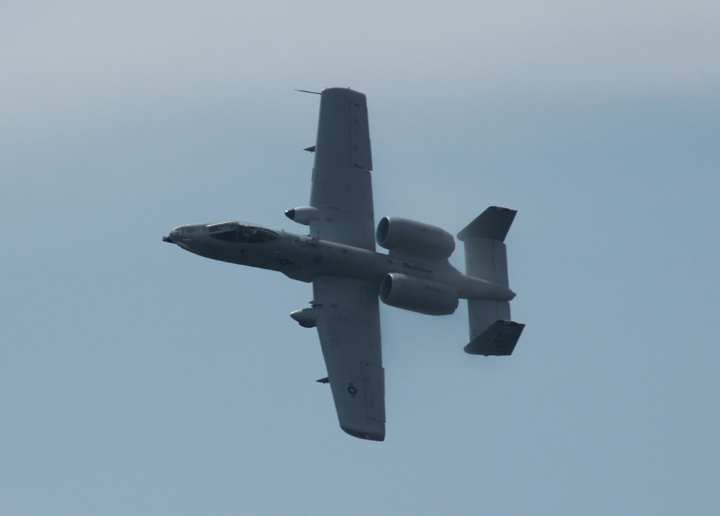Photo: Phil Medina, Jones Beach Memorial Day Air Show 2011
A10 Thunderbolt "Warthog" piloted by Major Thorpe.

An A10 Thunderbolt Warthog, piloted by Major Dylan "HABU" Thorpe, dives down for a strafing run on an enemy target at a velocity of 89 m/s. It fires its coke-bottle-sized bullets down its 3 meter cannon with an acceleration of 121,000 m/s2. What is the bullet’s velocity when it slams into its target?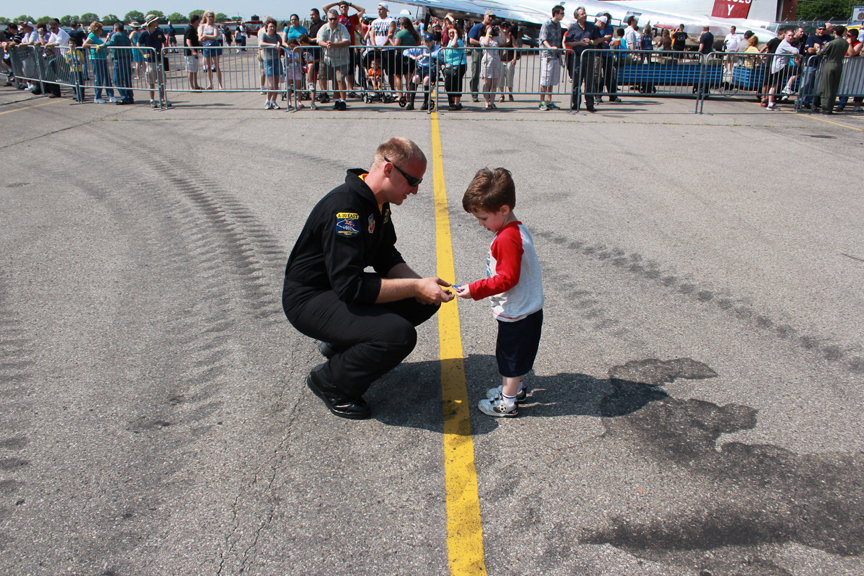My boy meeting Major Dylan "HABU" Thorpe.Me and my boy at the business end of a Warthog as Major Dylan "HABU" Thorpe looks on. *

## Equations of Motion

v = d/t
a = Δv/t
vf = vi + at
d = vit + ½at2

## Tips for Solving a Math problem

• Make a list of all known variables
• Identify what needs to be found
• Select an equation that will solve the problem
• Cross out whatever doesn’t apply (ex.: starting at rest)
• Rearrange your equation to solve for the unknown (algebra)
• Include units
• Check algebra by analyzing units
• Crunch the numbers

People solve equations out in the real world!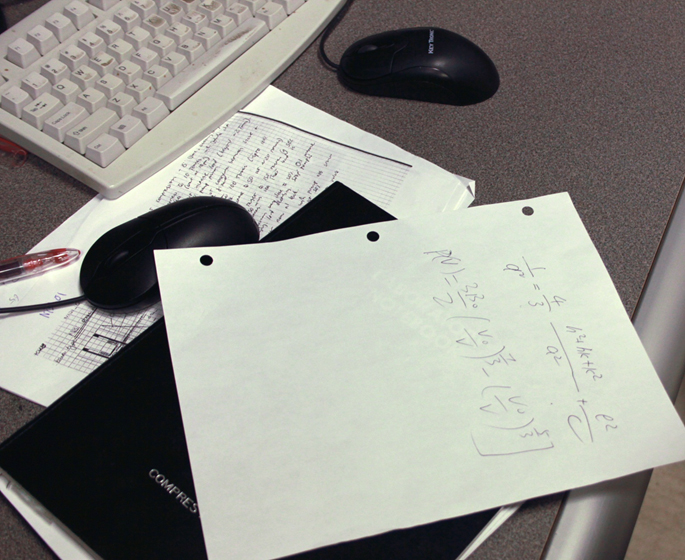A picture I took of a desk at Brookhaven National Lab during a tour.

### Don’t mix up the v’s

Each v means something different:

• [Average velocity]- ‘nuff said. This will not help you if you need to find the
beginning or ending velocities.
• vi: initial velocity- velocity at the beginning of the movement.
• vf: final velocity- velocity at the end of movement.
• Δv: change in velocity- the differenc ebetween the initial and final velocities or vf - vi

## On to VectorsMedina On-Line
Since 1994
Email: mrsciguy@optonline.net
 *All throughout the A10 Warthog demonstration at the Bethpage Air Show at Jones Beach State Park ™, the announcer continually said statements such as “and here comes Major Thorpe doing a strafing run” and “now Major Thorpe is demonstrating the A10’s tight cornering ability”. The announcer must have said "Major Thorpe" 50 times during the 10-minute demonstration- so apparently it is important. And the demonstration repeated at the end of the show with exactly the same maneuvers and commentary. I poke fun, if even for my own entertainment, at the announcer not Major Thorpe who I met and is a very nice man.